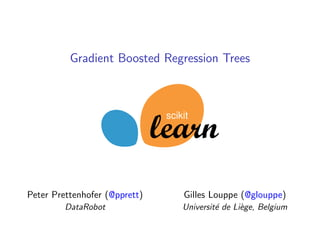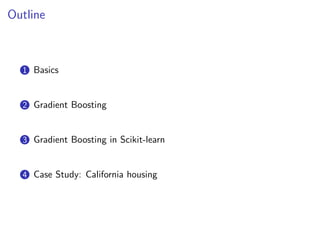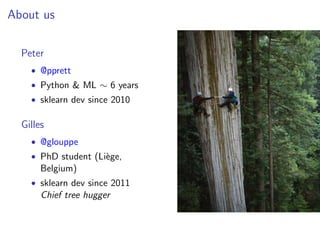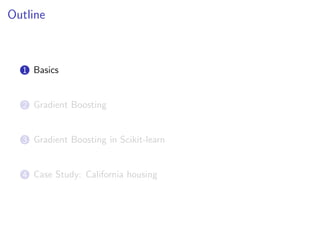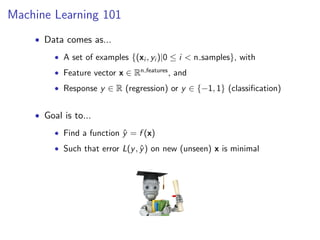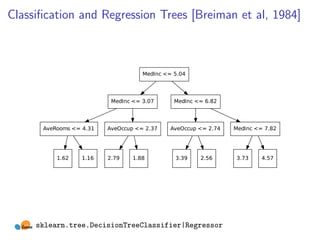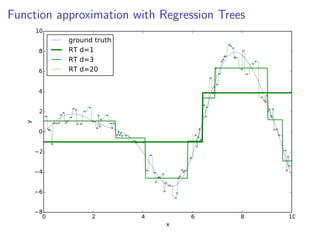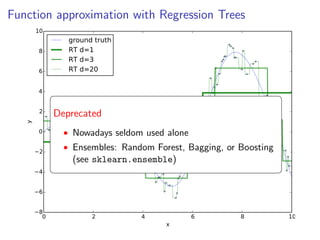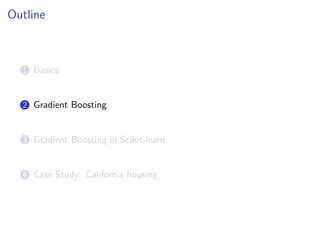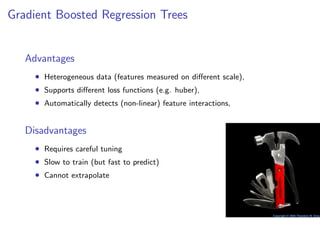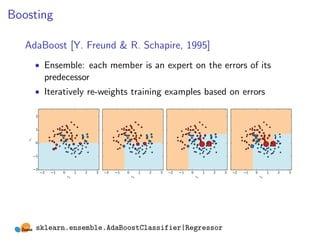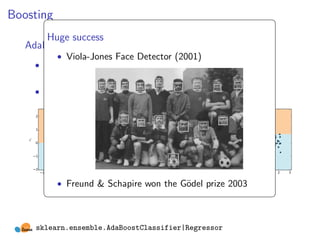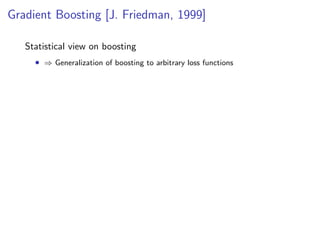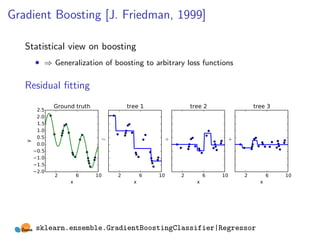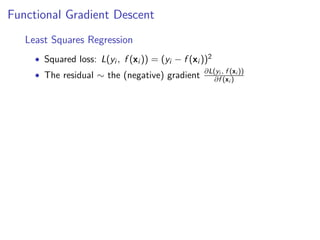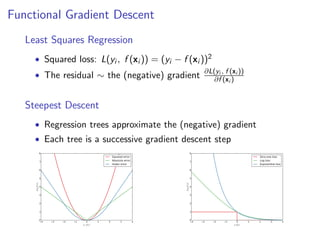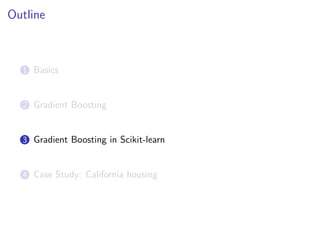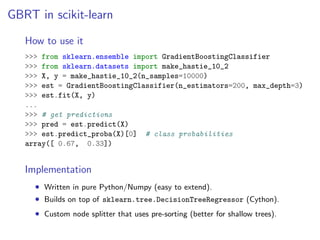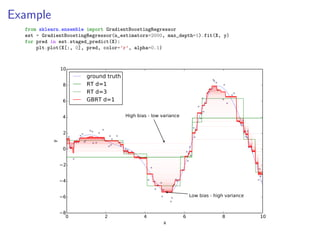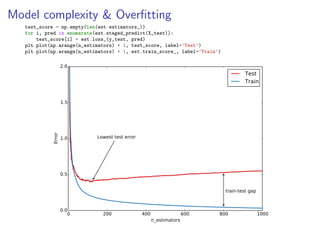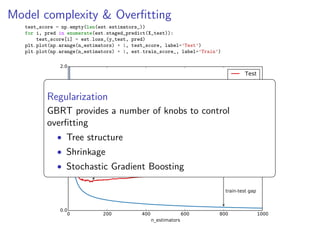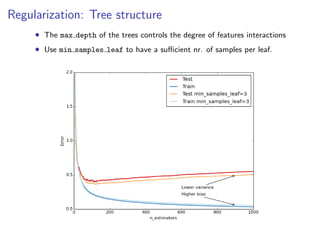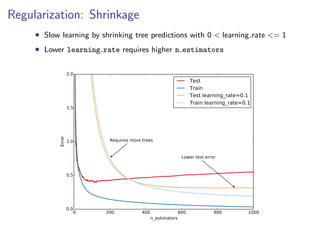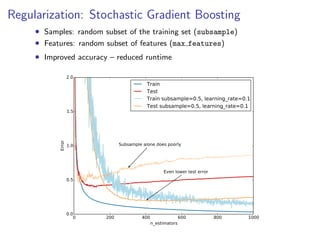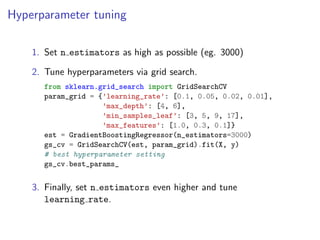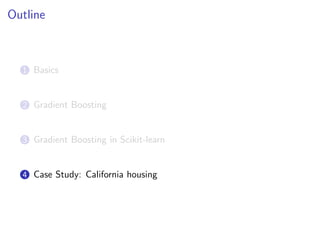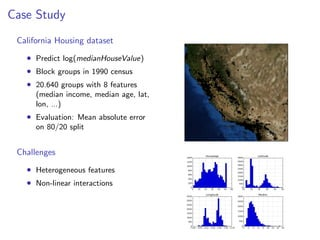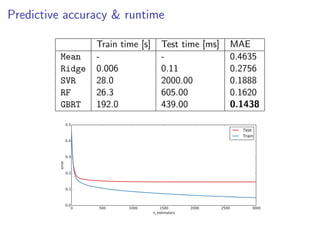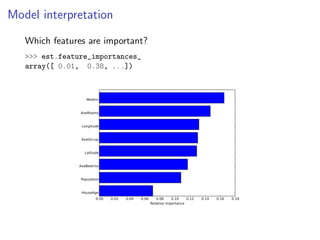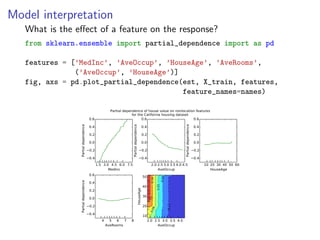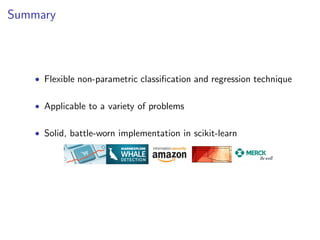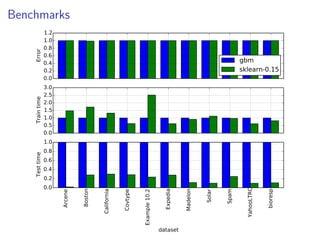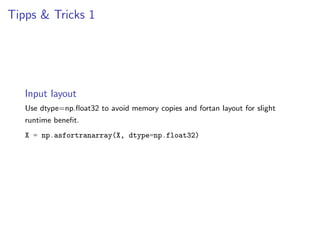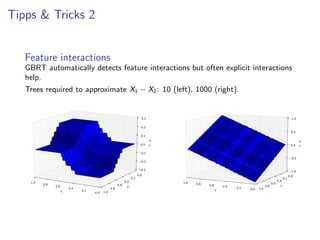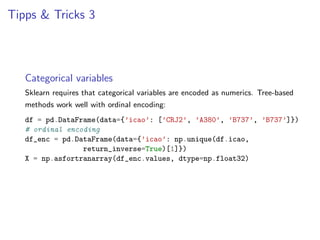1 of 39

### Gradient Boosted Regression Trees in scikit-learn

1. Gradient Boosted Regression Trees scikit Peter Prettenhofer (@pprett) Gilles Louppe (@glouppe) DataRobot Universit´ de Li`ge, Belgium e e
2. Motivation
3. Motivation
4. Outline 1 Basics 2 Gradient Boosting 3 Gradient Boosting in Scikit-learn 4 Case Study: California housing
5. About us Peter • @pprett • Python & ML ∼ 6 years • sklearn dev since 2010 Gilles • @glouppe • PhD student (Li`ge, e Belgium) • sklearn dev since 2011 Chief tree hugger
6. Outline 1 Basics 2 Gradient Boosting 3 Gradient Boosting in Scikit-learn 4 Case Study: California housing
7. Machine Learning 101 • Data comes as... • A set of examples {(xi , yi )|0 ≤ i < n samples}, with • Feature vector x ∈ Rn features , and • Response y ∈ R (regression) or y ∈ {−1, 1} (classiﬁcation) • Goal is to... • Find a function y = f (x) ˆ • Such that error L(y , y ) on new (unseen) x is minimal ˆ
8. Classiﬁcation and Regression Trees [Breiman et al, 1984] MedInc <= 5.04 MedInc <= 3.07 AveRooms <= 4.31 1.62 1.16 MedInc <= 6.82 AveOccup <= 2.37 AveOccup <= 2.74 2.79 1.88 3.39 2.56 sklearn.tree.DecisionTreeClassifier|Regressor MedInc <= 7.82 3.73 4.57
9. Function approximation with Regression Trees 10 8 6 ground truth RT d=1 RT d=3 RT d=20 4 y 2 0 2 4 6 8 0 2 4 x 6 8 10
10. Function approximation with Regression Trees 10 8 6 ground truth RT d=1 RT d=3 RT d=20 4 Deprecated y 2 0 • Nowadays seldom used alone 2 • Ensembles: Random Forest, Bagging, or Boosting (see sklearn.ensemble) 4 6 8 0 2 4 x 6 8 10
11. Outline 1 Basics 2 Gradient Boosting 3 Gradient Boosting in Scikit-learn 4 Case Study: California housing
12. Gradient Boosted Regression Trees Advantages • Heterogeneous data (features measured on diﬀerent scale), • Supports diﬀerent loss functions (e.g. huber), • Automatically detects (non-linear) feature interactions, Disadvantages • Requires careful tuning • Slow to train (but fast to predict) • Cannot extrapolate
13. Boosting AdaBoost [Y. Freund & R. Schapire, 1995] • Ensemble: each member is an expert on the errors of its predecessor • Iteratively re-weights training examples based on errors 2 x1 1 0 1 2 2 1 0 x0 1 2 3 2 1 0 x0 1 2 3 2 1 0 x0 1 2 sklearn.ensemble.AdaBoostClassifier|Regressor 3 2 1 0 x0 1 2 3
14. Boosting Huge success AdaBoost [Y. Freund & R. Schapire, 1995] • Viola-Jones Face Detector (2001) • Ensemble: each member is an expert on the errors of its predecessor • Iteratively re-weights training examples based on errors 2 x1 1 0 1 2 2 1 0 x0 1 2 3 2 1 0 x0 1 2 3 2 1 0 x0 1 2 3 2 1 • Freund & Schapire won the G¨del prize 2003 o sklearn.ensemble.AdaBoostClassifier|Regressor 0 x0 1 2 3
15. Gradient Boosting [J. Friedman, 1999] Statistical view on boosting • ⇒ Generalization of boosting to arbitrary loss functions
16. Gradient Boosting [J. Friedman, 1999] Statistical view on boosting • ⇒ Generalization of boosting to arbitrary loss functions y Residual ﬁtting 2.5 2.0 1.5 1.0 0.5 0.0 0.5 1.0 1.5 2.0 Ground truth tree 1 + ∼ 2 x 6 10 tree 2 2 x 6 10 tree 3 + 2 x 6 10 2 sklearn.ensemble.GradientBoostingClassifier|Regressor x 6 10
17. Functional Gradient Descent Least Squares Regression • Squared loss: L(yi , f (xi )) = (yi − f (xi ))2 f • The residual ∼ the (negative) gradient ∂L(yi ,(x (xi )) ∂f i )
18. Functional Gradient Descent Least Squares Regression • Squared loss: L(yi , f (xi )) = (yi − f (xi ))2 f • The residual ∼ the (negative) gradient ∂L(yi ,(x (xi )) ∂f i ) Steepest Descent • Regression trees approximate the (negative) gradient • Each tree is a successive gradient descent step 8 8 Squared error Absolute error Huber error 7 6 6 5 L(y,f(x)) 5 L(y,f(x)) Zero-one loss Log loss Exponential loss 7 4 4 3 3 2 2 1 0 1 4 3 2 1 0 y−f(x) 1 2 3 4 0 4 3 2 1 0 y ·f(x) 1 2 3 4
19. Outline 1 Basics 2 Gradient Boosting 3 Gradient Boosting in Scikit-learn 4 Case Study: California housing
20. GBRT in scikit-learn How to use it >>> from sklearn.ensemble import GradientBoostingClassifier >>> from sklearn.datasets import make_hastie_10_2 >>> X, y = make_hastie_10_2(n_samples=10000) >>> est = GradientBoostingClassifier(n_estimators=200, max_depth=3) >>> est.fit(X, y) ... >>> # get predictions >>> pred = est.predict(X) >>> est.predict_proba(X) # class probabilities array([ 0.67, 0.33]) Implementation • Written in pure Python/Numpy (easy to extend). • Builds on top of sklearn.tree.DecisionTreeRegressor (Cython). • Custom node splitter that uses pre-sorting (better for shallow trees).
21. Example from sklearn.ensemble import GradientBoostingRegressor est = GradientBoostingRegressor(n_estimators=2000, max_depth=1).fit(X, y) for pred in est.staged_predict(X): plt.plot(X[:, 0], pred, color=’r’, alpha=0.1) 10 8 6 ground truth RT d=1 RT d=3 GBRT d=1 High bias - low variance 4 y 2 0 2 4 Low bias - high variance 6 8 0 2 4 x 6 8 10
22. Model complexity & Overﬁtting test_score = np.empty(len(est.estimators_)) for i, pred in enumerate(est.staged_predict(X_test)): test_score[i] = est.loss_(y_test, pred) plt.plot(np.arange(n_estimators) + 1, test_score, label=’Test’) plt.plot(np.arange(n_estimators) + 1, est.train_score_, label=’Train’) 2.0 Test Train Error 1.5 1.0 Lowest test error 0.5 train-test gap 0.0 0 200 400 n_estimators 600 800 1000
23. Model complexity & Overﬁtting test_score = np.empty(len(est.estimators_)) for i, pred in enumerate(est.staged_predict(X_test)): test_score[i] = est.loss_(y_test, pred) plt.plot(np.arange(n_estimators) + 1, test_score, label=’Test’) plt.plot(np.arange(n_estimators) + 1, est.train_score_, label=’Train’) 2.0 Test Train Regularization 1.5 GBRT provides a number of knobs to control overﬁtting Error Lowest test •1.0Tree structure error • Shrinkage • Stochastic Gradient Boosting 0.5 train-test gap 0.0 0 200 400 n_estimators 600 800 1000
24. Regularization: Tree structure • The max depth of the trees controls the degree of features interactions • Use min samples leaf to have a suﬃcient nr. of samples per leaf.
25. Regularization: Shrinkage • Slow learning by shrinking tree predictions with 0 < learning rate <= 1 • Lower learning rate requires higher n estimators 2.0 Test Train Test learning_rate=0.1 Train learning_rate=0.1 Error 1.5 1.0 Requires more trees Lower test error 0.5 0.0 0 200 400 n_estimators 600 800 1000
26. Regularization: Stochastic Gradient Boosting • Samples: random subset of the training set (subsample) • Features: random subset of features (max features) • Improved accuracy – reduced runtime 2.0 Train Test Train subsample=0.5, learning_rate=0.1 Test subsample=0.5, learning_rate=0.1 Error 1.5 Subsample alone does poorly 1.0 Even lower test error 0.5 0.0 0 200 400 n_estimators 600 800 1000
27. Hyperparameter tuning 1. Set n estimators as high as possible (eg. 3000) 2. Tune hyperparameters via grid search. from sklearn.grid_search import GridSearchCV param_grid = {’learning_rate’: [0.1, 0.05, 0.02, 0.01], ’max_depth’: [4, 6], ’min_samples_leaf’: [3, 5, 9, 17], ’max_features’: [1.0, 0.3, 0.1]} est = GradientBoostingRegressor(n_estimators=3000) gs_cv = GridSearchCV(est, param_grid).fit(X, y) # best hyperparameter setting gs_cv.best_params_ 3. Finally, set n estimators even higher and tune learning rate.
28. Outline 1 Basics 2 Gradient Boosting 3 Gradient Boosting in Scikit-learn 4 Case Study: California housing
29. Case Study California Housing dataset • Predict log(medianHouseValue) • Block groups in 1990 census • 20.640 groups with 8 features (median income, median age, lat, lon, ...) • Evaluation: Mean absolute error on 80/20 split Challenges • Heterogeneous features • Non-linear interactions
30. Predictive accuracy & runtime Mean Ridge SVR RF GBRT Train time [s] 0.006 28.0 26.3 192.0 Test time [ms] 0.11 2000.00 605.00 439.00 MAE 0.4635 0.2756 0.1888 0.1620 0.1438 0.5 Test Train 0.4 error 0.3 0.2 0.1 0.0 0 500 1000 1500 n_estimators 2000 2500 3000
31. Model interpretation Which features are important? >>> est.feature_importances_ array([ 0.01, 0.38, ...]) MedInc AveRooms Longitude AveOccup Latitude AveBedrms Population HouseAge 0.00 0.02 0.04 0.06 0.08 0.10 0.12 Relative importance 0.14 0.16 0.18
32. Model interpretation What is the eﬀect of a feature on the response? from sklearn.ensemble import partial_dependence import as pd Partial dependence -0.12 0.09 0.2 3 0.02 0.16 -0.05 Partial dependence Partial dependence of house value on nonlocation features for the California housing dataset 0.6 0.6 0.4 0.4 0.2 0.2 0.0 0.0 0.2 0.2 0.4 0.4 1.5 3.0 4.5 6.0 7.5 2.0 2.5 3.0 3.5 4.0 4.5 10 20 30 40 50 60 MedInc AveOccup HouseAge 0.6 50 0.4 40 0.2 30 0.0 20 0.2 0.4 10 4 5 6 7 8 2.0 2.5 3.0 3.5 4.0 AveRooms AveOccup 0.6 0.4 0.2 0.0 0.2 0.4 HouseAge Partial dependence Partial dependence features = [’MedInc’, ’AveOccup’, ’HouseAge’, ’AveRooms’, (’AveOccup’, ’HouseAge’)] fig, axs = pd.plot_partial_dependence(est, X_train, features, feature_names=names)
33. Model interpretation Automatically detects spatial eﬀects 0.97 0.57 0.66 0.49 0.41 partial dep. on median house value partial dep. on median house value 0.34 0.33 -0.28 0.25 latitude latitude 0.03 0.17 -0.60 0.09 -0.91 0.01 -0.07 -1.22 longitude -1.54 -0.15 longitude
34. Summary • Flexible non-parametric classiﬁcation and regression technique • Applicable to a variety of problems • Solid, battle-worn implementation in scikit-learn
35. Thanks! Questions?
36. Test time Train time Error 1.2 1.0 0.8 0.6 0.4 0.2 0.0 3.0 2.5 2.0 1.5 1.0 0.5 0.0 1.0 0.8 0.6 0.4 0.2 0.0 dataset bioresp YahooLTRC Spam Solar Madelon Expedia Example 10.2 Covtype California Boston Arcene Benchmarks gbm sklearn-0.15
37. Tipps & Tricks 1 Input layout Use dtype=np.ﬂoat32 to avoid memory copies and fortan layout for slight runtime beneﬁt. X = np.asfortranarray(X, dtype=np.float32)
38. Tipps & Tricks 2 Feature interactions GBRT automatically detects feature interactions but often explicit interactions help. Trees required to approximate X1 − X2 : 10 (left), 1000 (right). 0.3 1.0 0.2 x-y 0.0 0.0 0.1 0.5 0.2 1.0 0.8 0.6 x 0.4 0.2 0.0 1.0 0.8 0.6 0.4 y 0.2 x-y 0.5 0.1 0.3 0.0 1.0 0.8 0.6 x 0.4 0.2 0.0 1.0 0.8 0.6 0.4 y 0.2 1.0 0.0
39. Tipps & Tricks 3 Categorical variables Sklearn requires that categorical variables are encoded as numerics. Tree-based methods work well with ordinal encoding: df = pd.DataFrame(data={’icao’: [’CRJ2’, ’A380’, ’B737’, ’B737’]}) # ordinal encoding df_enc = pd.DataFrame(data={’icao’: np.unique(df.icao, return_inverse=True)}) X = np.asfortranarray(df_enc.values, dtype=np.float32)